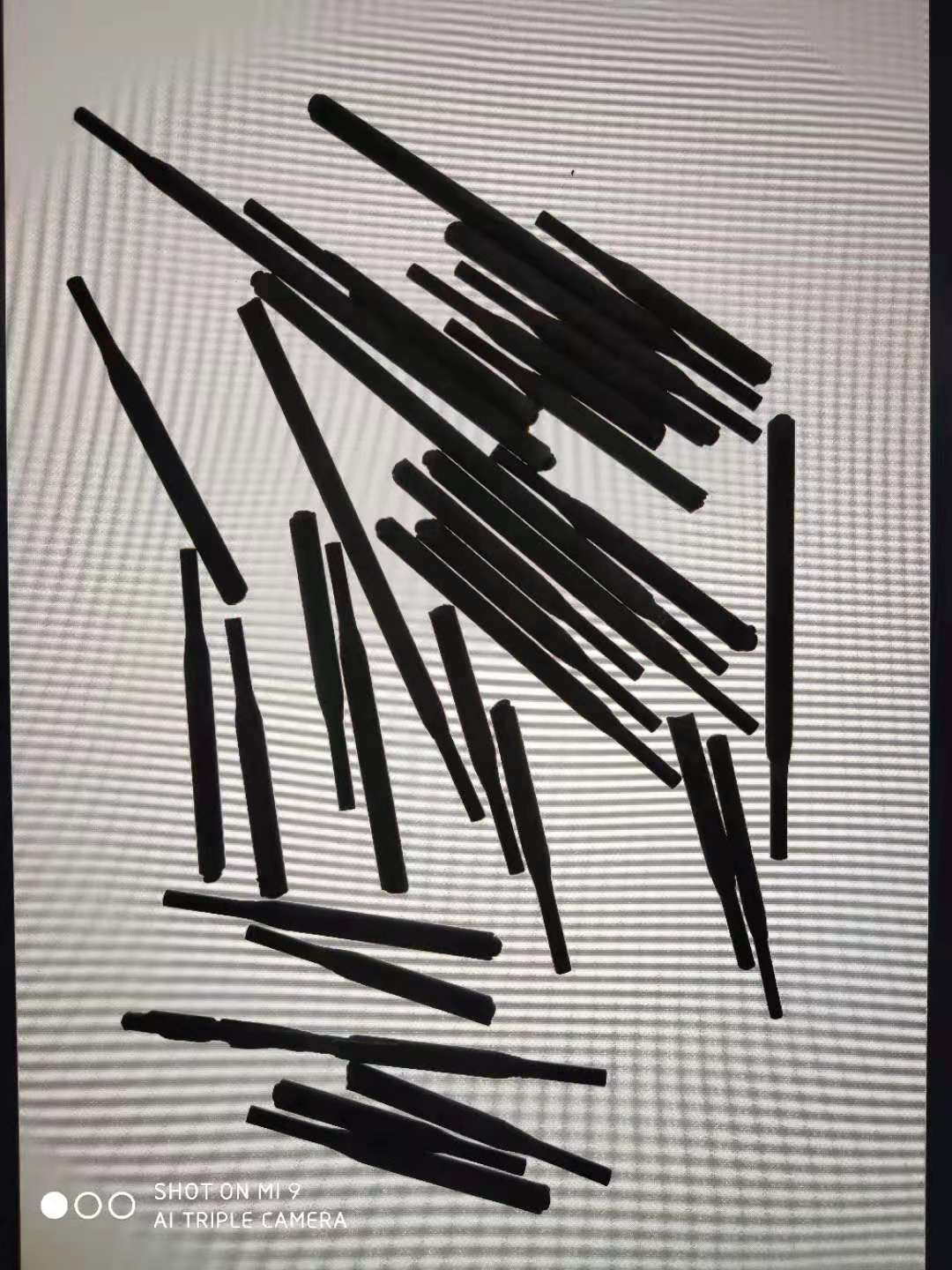2021-08-03 17:29

# opencv计数项目实现及步骤• 点赞
• 收藏

#### 2条回答默认 最新

•王大师王文峰 2021-08-03 17:59
已采纳

opencv计数项目实现代码

``````#include<iostream>
#include<opencv.hpp>
using namespace std;
using namespace cv;

int main()
{
//加载图像
if (src.empty())
{
cout << "no image!" << endl;
return -1;
}
imshow("src", src);
//二值化
Mat gray, binary;
cvtColor(src, gray, COLOR_BGR2GRAY);
imshow("gray", gray);
threshold(gray, binary, 0, 255, THRESH_TRIANGLE);
imshow("binary", binary);
//图像形态学操作
Mat kern1 = getStructuringElement(MORPH_RECT, Size(5, 5), Point(-1,-1));
morphologyEx(binary, binary, MORPH_DILATE, kern1,Point(-1,-1),3);
imshow("dilate", binary);
//距离变换
Mat dist;
bitwise_not(binary, binary);//将白色背景变黑
imshow("not", binary);
distanceTransform(binary, dist, DIST_L2, 3);
normalize(dist, dist, 0.0, 1.0, NORM_MINMAX);
dist.convertTo(dist, CV_8UC1);
imshow("dist", dist);
//阈值分割
Mat img;
//threshold(dist, img, 0, 255, THRESH_BINARY | THRESH_OTSU);
imshow("img", img);
//腐蚀操作
morphologyEx(img, img, MORPH_DILATE, kern1, Point(-1,-1),1);
imshow("dilate-img", img);
//寻找连通域
vector<vector<Point>>contours;
findContours(img, contours, RETR_EXTERNAL, CHAIN_APPROX_SIMPLE);
for (size_t i = 0; i < contours.size(); i++)
{
drawContours(mask, contours, static_cast<int>(i), Scalar(0, 0, 255), -1, 8);
}
cout << "个数：" << contours.size() << endl;

waitKey(0);
return 0;
}

``````
点赞 打赏 评论
•不吐西瓜籽 2021-08-03 17:55

这个和人眼识别是一样的，需要先确认检测物品然后计数，将已识别物品标记去掉，防止循环识别，将整张图过滤完后就可以输出计数了

点赞 打赏 评论